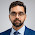Equivalent circulating density-ECD

Equivalent circulating density is the density of drilling fluid during circulation.Actually mud density remains the same under static or circulating condition but the pressure under both the condition differs.When the drilling mud is under static condition(no circulation),Pressure at any point is only due to drilling mud weight and is given by:-

Pressure under static Condition = 0.052* Mud weight(in ppg)* TVD(in feet)(eq-1)

But during circulation, extra pressure is applied by the mud pumps to circulate the drilling fluid. This pump pressure is equal to the total pressure loss in the circulating system.That’s why during circulation, pressure at any point in the well-bore will be due to mud weight plus the pressure loss in the system.

Circulating pressure = Pressure under static Condition + pressure loss in the system

ECD is always calculated for annulus as it has no importance inside the drill string that's why we can replace pressure loss in the system with annular pressure loss.

Circulating pressure in the annulus = Pressure under static Condition+ Annular pressure loss (eq-2)
ECD can also be defined as the density equivalent of circulating pressure in the annulus.
Circulating pressure in the annulus= 0.052* ECD* TVD(eq-3)
Putting equation-3 in equation-2 we get:-
0.052* TVD* ECD= Pressure under static Condition + Annular pressure loss
ECD=(Pressure under static condition + Annular pressure loss) / (0.052* TVD)

Importance of ECD:-

For safe drilling it is must to keep the ECD lower than the formation fracture gradient otherwise the formation will fracture that's why ECD is continuously monitored while drilling.

ECD < Fracture pressure gradient, to avoid fracturing the formation
Mud weight >=Pore pressure, to avoid kick

Note:-During circulation ECD will always be greater than or equal to Mud weight.

1.2.